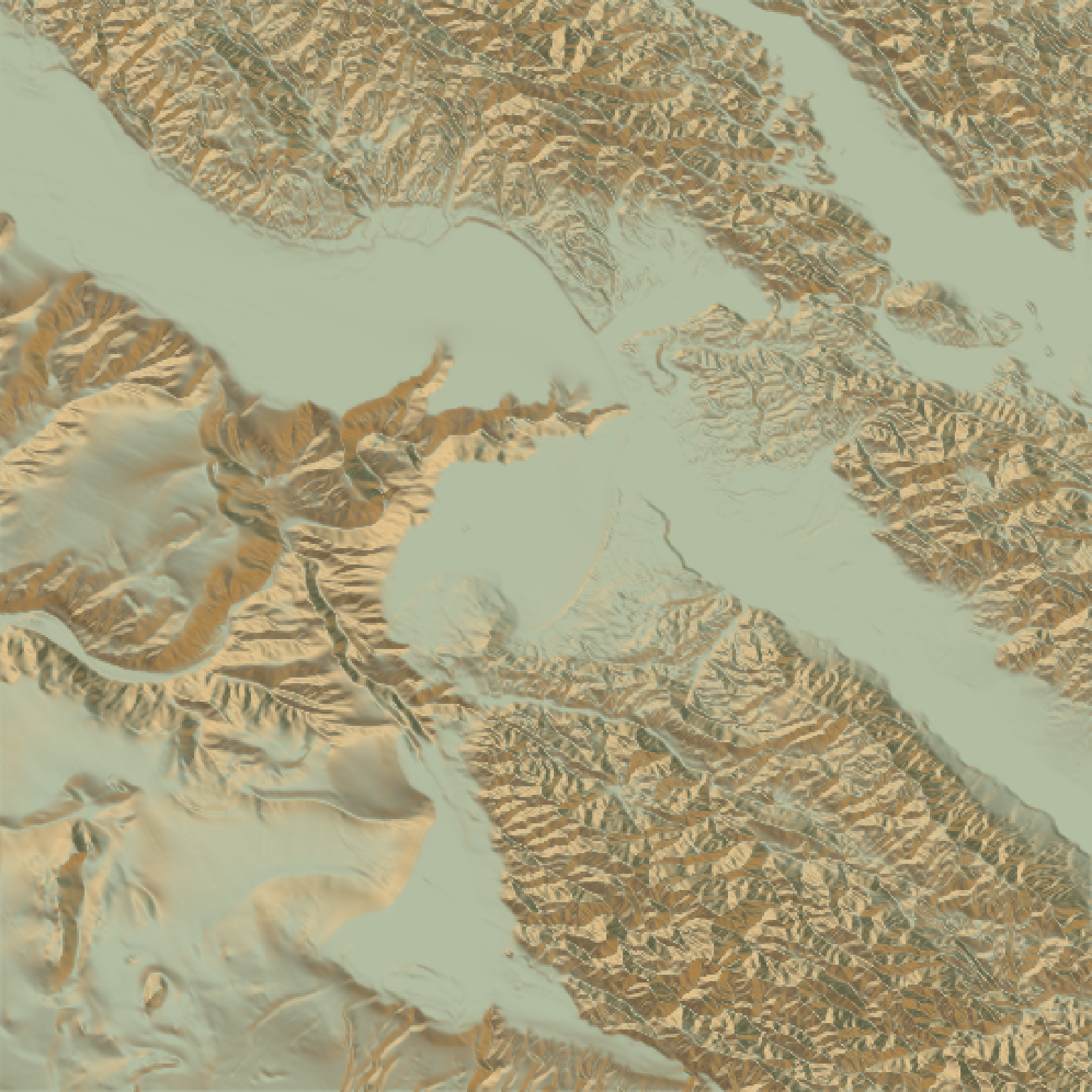Calculates a color for each point on the surface using the surface normals and hemispherical UV mapping. This uses either a texture map provided by the user (as an RGB array), or a built-in color texture.

sphere_shade(
heightmap,
sunangle = 315,
texture = "imhof1",
normalvectors = NULL,
colorintensity = 1,
zscale = 1,
progbar = interactive()
)

## Arguments

heightmap A two-dimensional matrix, where each entry in the matrix is the elevation at that point. All points are assumed to be evenly spaced. Default 315 (NW). The direction of the main highlight color (derived from the built-in palettes or the create_texture function). Default imhof1. Either a square matrix indicating the spherical texture mapping, or a string indicating one of the built-in palettes (imhof1,imhof2,imhof3,imhof4,desert, bw, and unicorn). Default NULL. Cache of the normal vectors (from calculate_normal function). Supply this to speed up texture mapping. Default 1. The intensity of the color mapping. Higher values will increase the intensity of the color mapping. Default 1/colorintensity. The ratio between the x and y spacing (which are assumed to be equal) and the z axis. Ignored unless colorintensity missing. Default TRUE if interactive, FALSE otherwise. If FALSE, turns off progress bar.

## Value

RGB array of hillshaded texture mappings.

## Examples

#Basic example:
montereybay %>%
plot_map()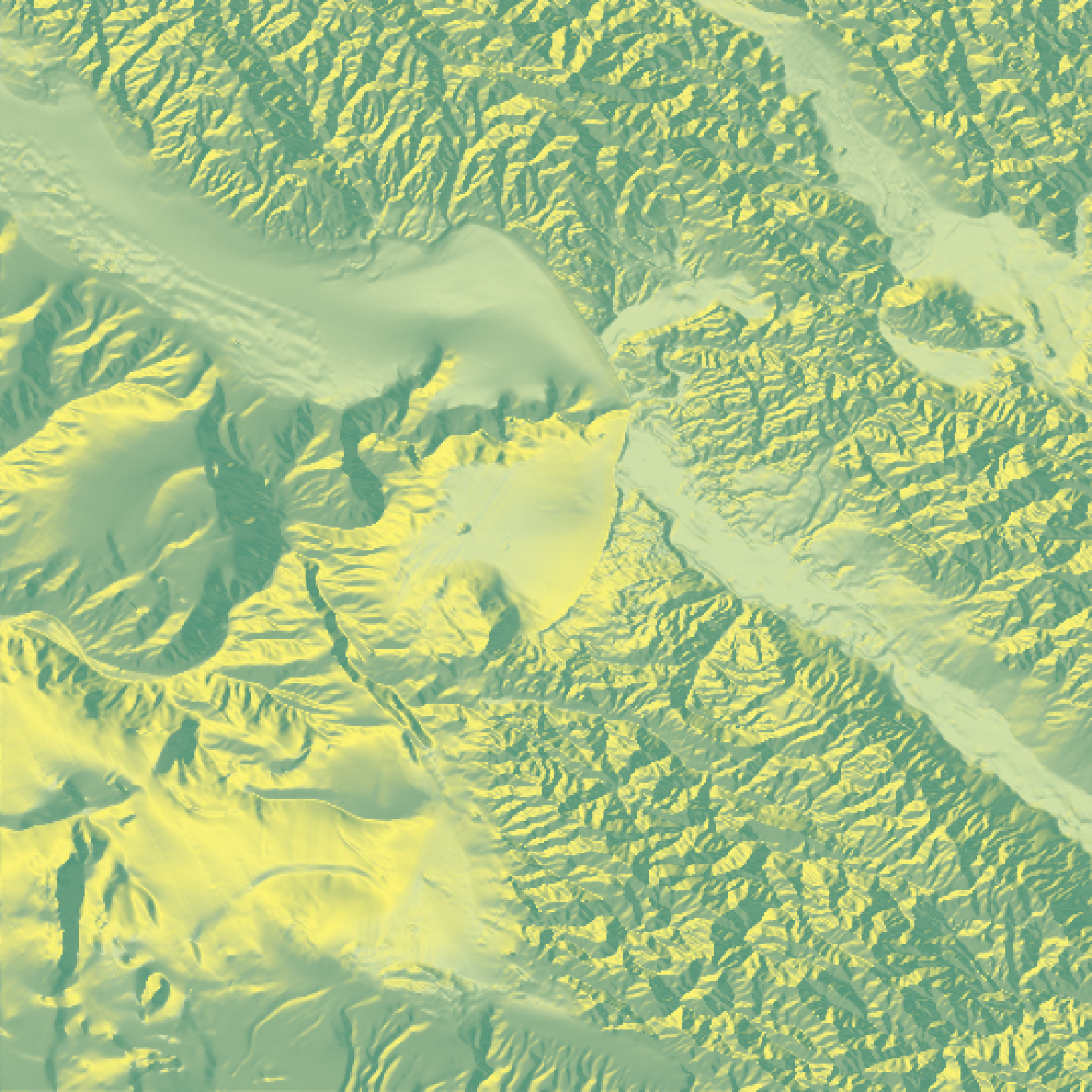#Decrease the color intensity:
montereybay %>%
plot_map()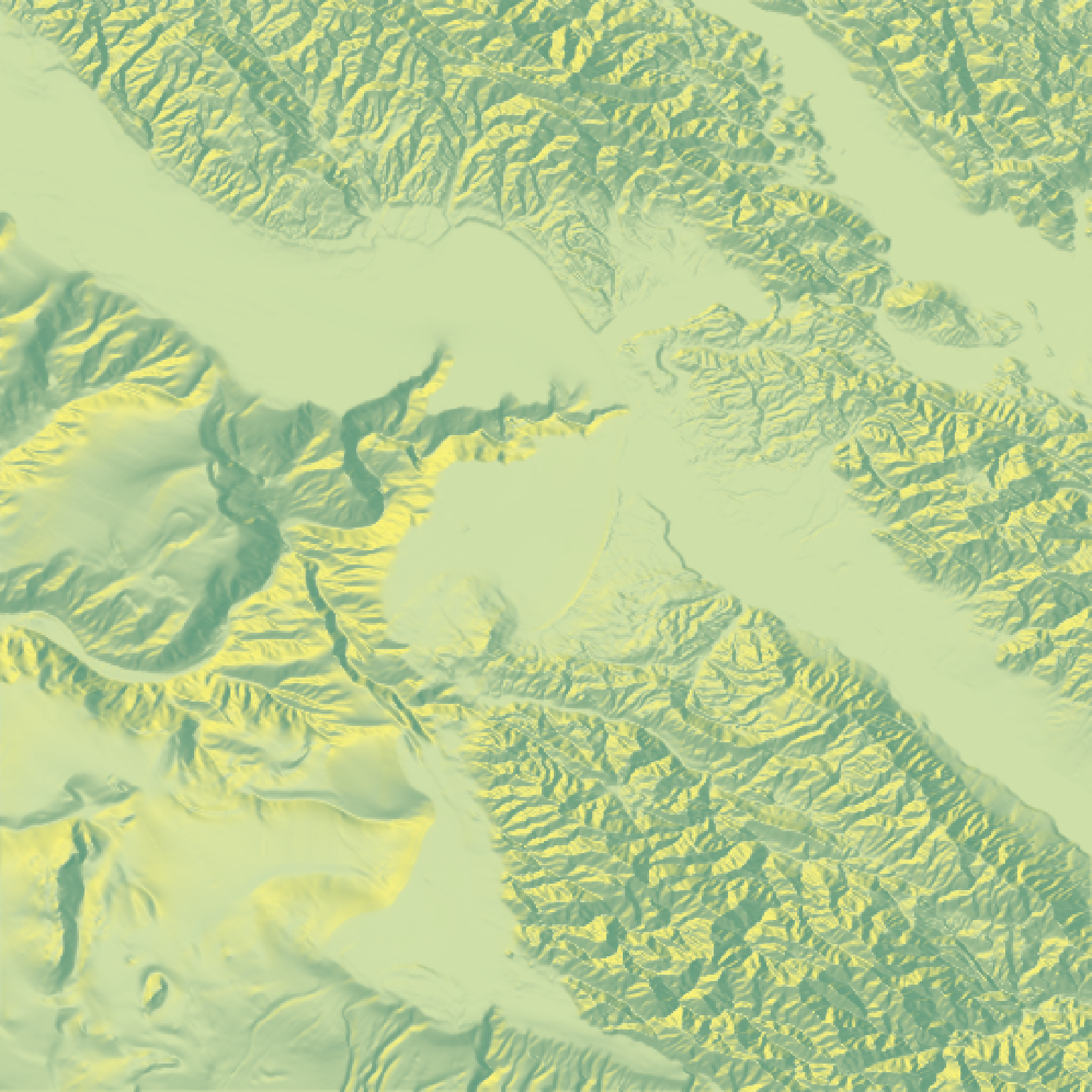#Change to a built-in color texture:
montereybay %>%
plot_map()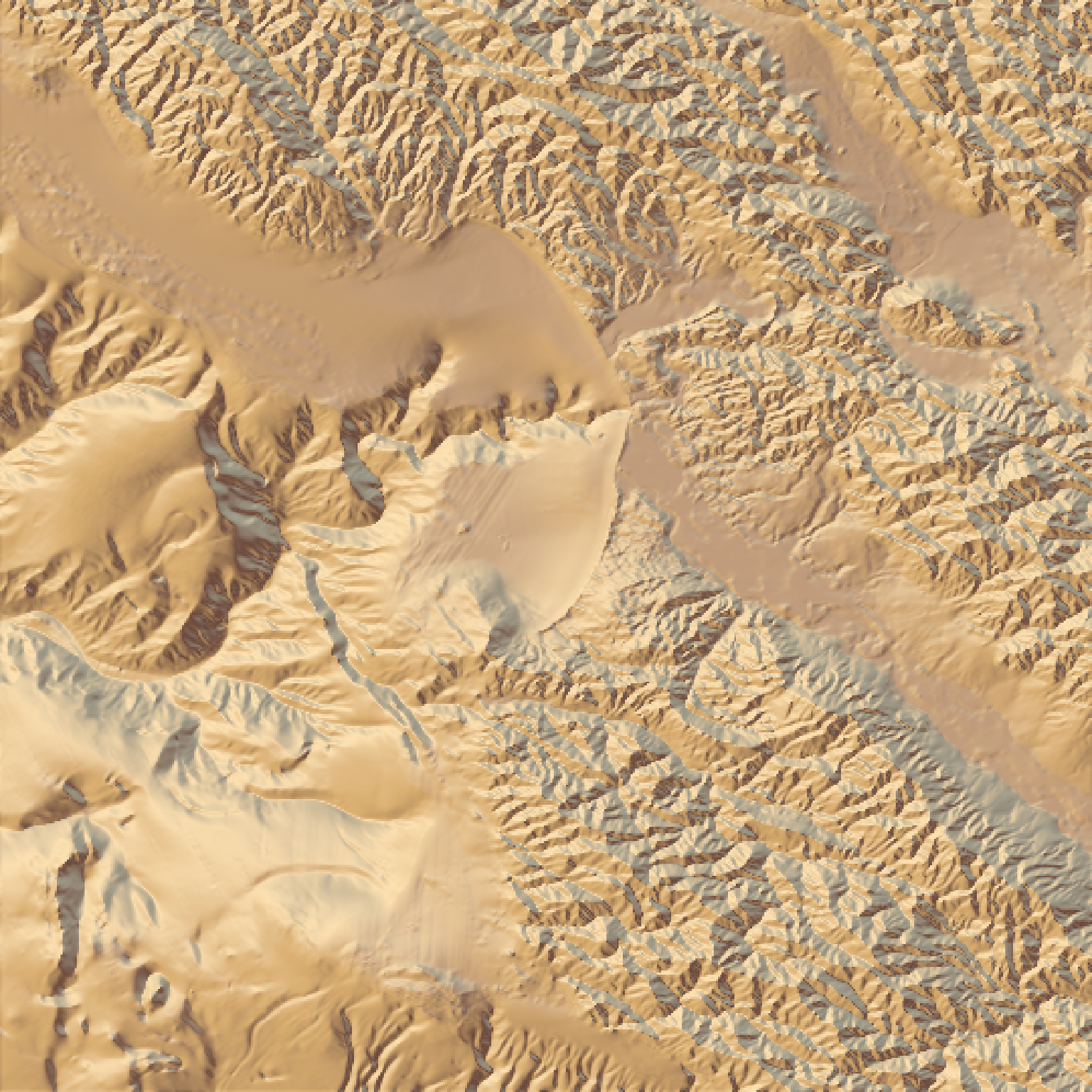plot_map()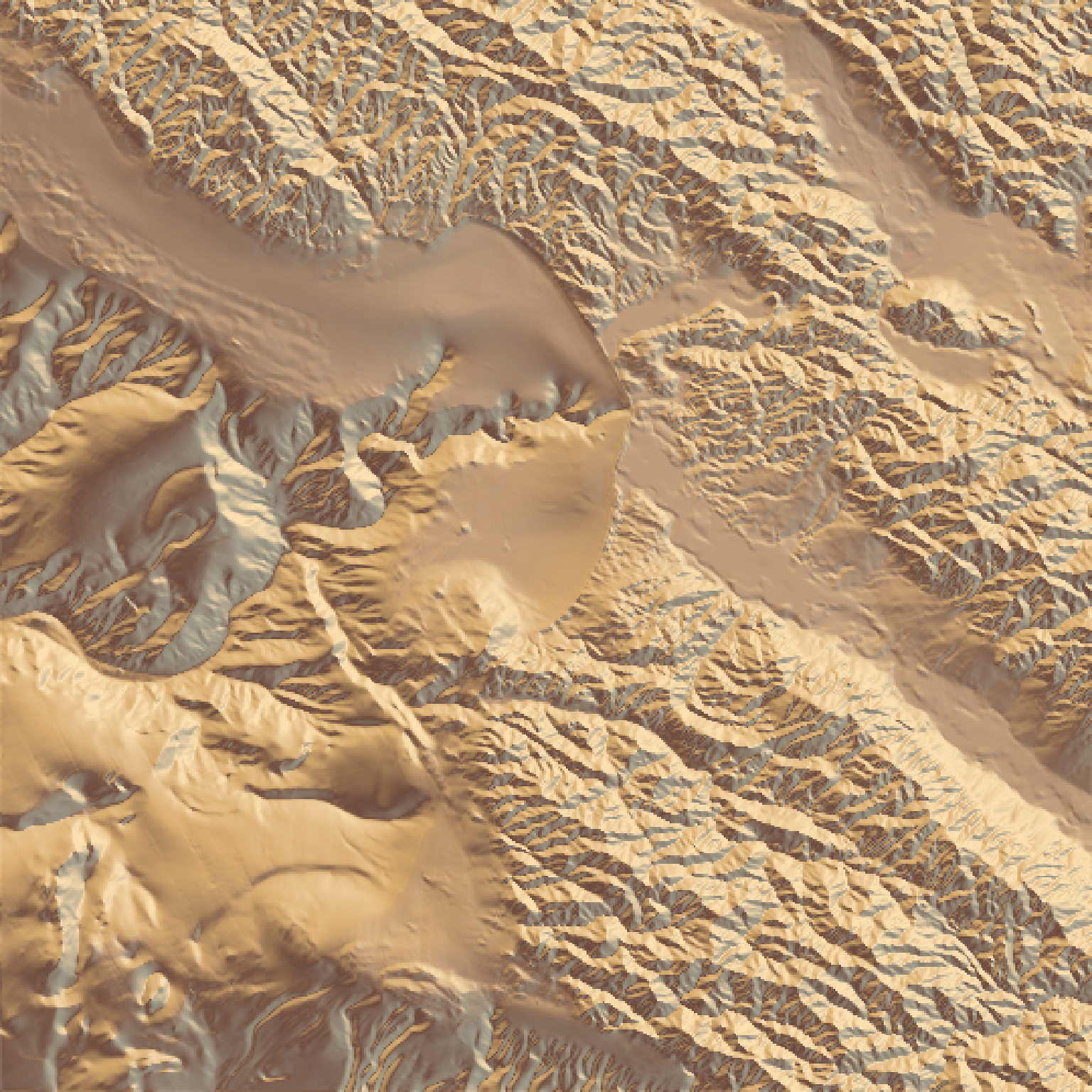#Create our own texture using the create_texture function:
plot_map()